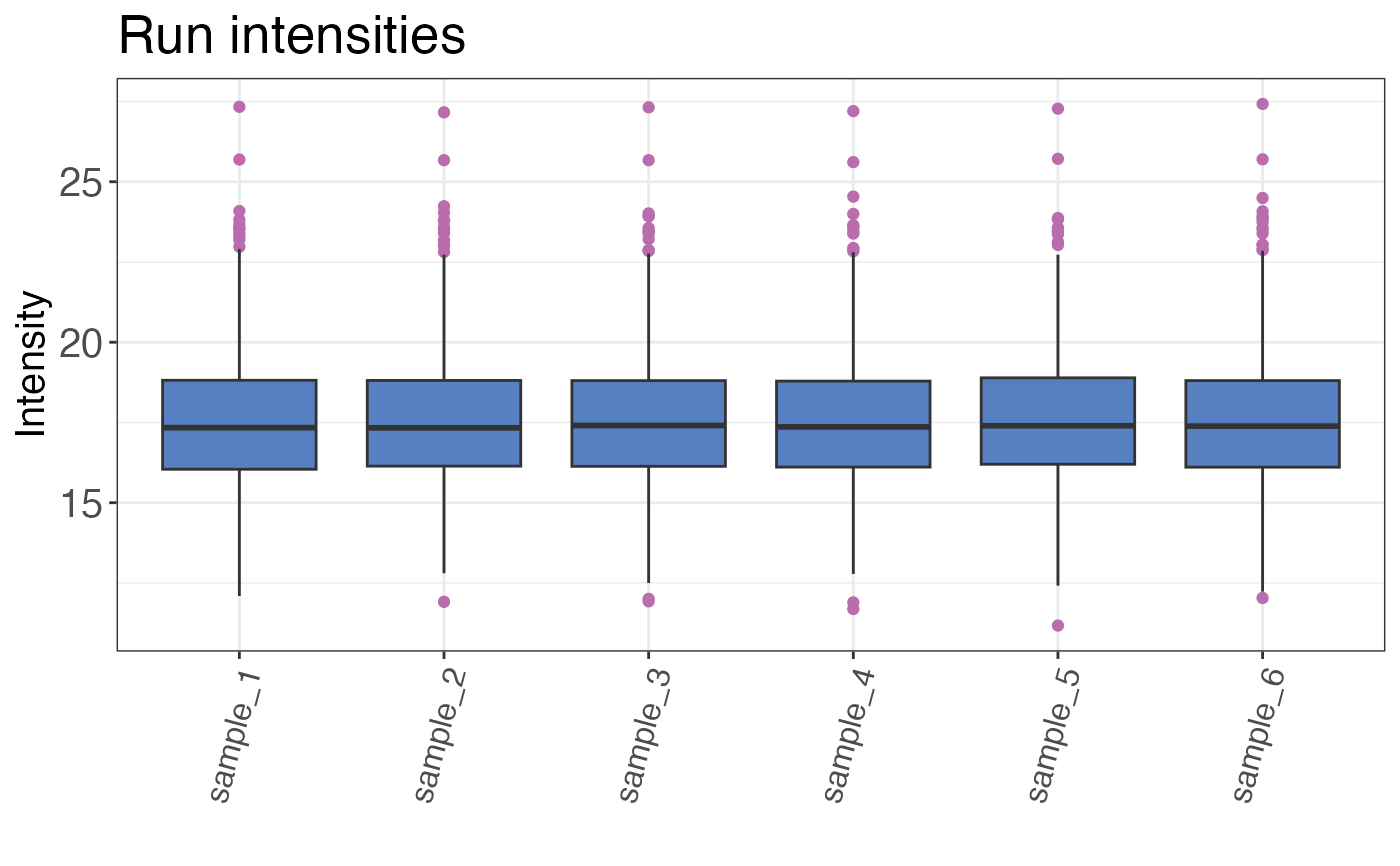Plots the overall or sample-wise distribution of all peptide intensities as a boxplot or histogram.

qc_intensity_distribution(
data,
sample = NULL,
grouping,
intensity_log2,
plot_style
)

## Arguments

data a data frame that contains at least sample names, grouping identifiers (precursor, peptide or protein) and log2 transformed intensities for each grouping identifier. a character column in the data data frame that contains the sample name. NOTE: If the overall distribution should be returned please do not provide the name of the sample column. a character column in the data data frame that contains the grouping variables (e.g. peptides, precursors or proteins). a numeric column in the data data frame that contains the log2 transformed intensities of each grouping identifier sample combination. a character value that indicates the plot type. This can be either "histogram", "boxplot" or "violin". Plot style "boxplot" and "violin" can only be used if a sample column is provided.

## Value

A histogram or boxplot that shows the intensity distribution over all samples or by sample.

## Examples

set.seed(123) # Makes example reproducible

# Create example data
data <- create_synthetic_data(
n_proteins = 100,
frac_change = 0.05,
n_replicates = 3,
n_conditions = 2,
method = "effect_random"
)

# Plot intensity distribution
# The plot style can be changed
qc_intensity_distribution(
data = data,
sample = sample,
grouping = peptide,
intensity_log2 = peptide_intensity_missing,
plot_style = "boxplot"
)